Definitions of Square Dance Calls and Concepts

\$BM?\$(\$i\$l\$?%3!<%k\$r9=@.MWAG\$N%Q!<%H\$KJ,2r\$7\$F(B, \$B\$=\$N%Q!<%H\$r5U\$N=g=x\$G9T\$\$\$^\$9(B.

\$BCalls With Parts.

Reverse Order Recoil: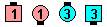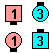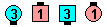Reverse Order Recoil\$B\$NA0(B Step & Fold\$B\$N8e(B Box Recycle\$B\$N8e(B (\$B=*\$o\$j(B)
Reverse Order Travel Thru: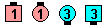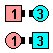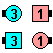Reverse Order Travel Thru\$B\$NA0(B As Couples 1/4 Right\$B\$N8e(B Pass Thru\$B\$N8e(B (\$B=*\$o\$j(B)
Reverse Order Diamond Chain Thru: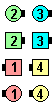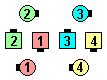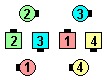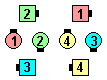Reverse Order Diamond Chain Thru\$B\$NA0(B Centers Cast Off 3/4\$B\$N8e(B Very Centers Trade\$B\$N8e(B Diamond Circulate\$B\$N8e(B (\$B=*\$o\$j(B)

\$BCmReverse Order \$B\$r0UL#\$9\$k\$H\$-\$K\$H\$-\$K(B, \$BC1\$K(B Reverse \$B\$H8@\$\$\$^\$9(B. \$B\$3\$l\$O(B, \$BB?\$/\$N>l9g\$"\$\$\$^\$\$\$G\$9(B. Reverse Recoil \$B\$r9M\$(\$F\$_\$k\$H(B: \$B%3!<%i!<\$O(B, Reverse (Mirror) Recycle \$B\$r\$7\$F(B Step & Fold \$B\$r\$7\$?\$\$\$N\$+(B, \$B\$^\$?\$O(B Step & Fold \$B\$r\$7\$F(B Split Recycle \$B\$r\$7\$?\$\$\$N\$+(B, \$BJ,\$+\$j\$^\$;\$s(B.

\$B \$B

• Reverse Order Split Turn The Key:
Hinge; Split Counter Rotate 1/4; Trade. Columns \$B\$G=*\$o\$j\$^\$9(B.
• Split Reverse Order Turn The Key:
Hinge; Lockit; Trade. Tidal Wave \$B\$G=*\$o\$j\$^\$9(B.

\$B8eSplit \$B\$N8@MU\$r@h\$KJ9\$\$\$F\$\$\$k\$N\$G(B, \$B;O\$a\$N(B formation \$B\$r(B 2 x 2 \$B\$XJ,\$1\$J\$1\$l\$P\$J\$j\$^\$;\$s(B. \$B\$=\$7\$F(B, 2 x 2 \$B\$N3F!9\$G%3!<%k\$r9T\$\$\$^\$9(B.

\$BReverse The Top [C3B] \$B\$=\$l\$O(B, Reverse Order Spin The Top \$B\$G\$9(B.